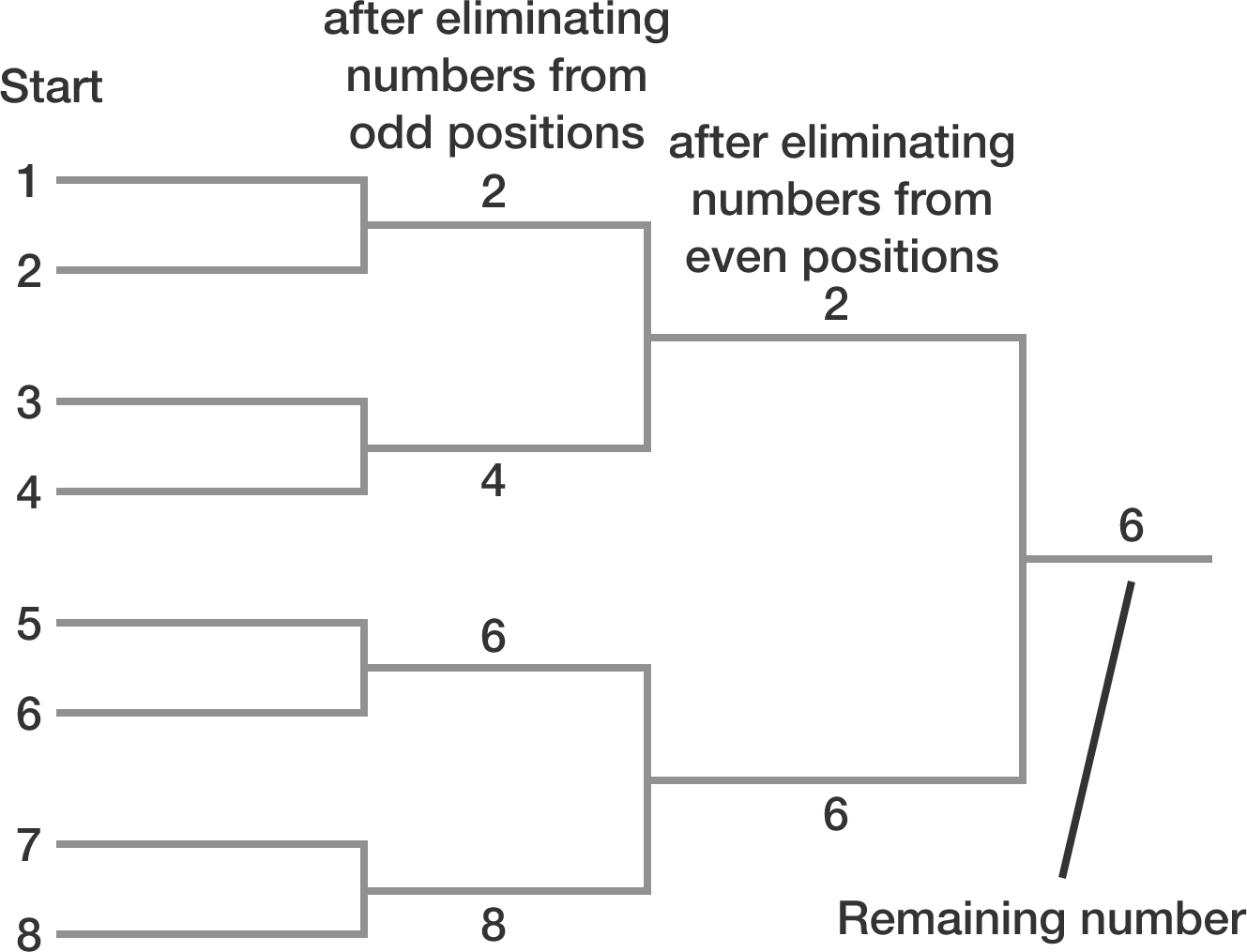# Odd-Even elimination

The integers 1 through 12345 (inclusive) are written in a row in ascending order. You and your friend take turns removing numbers according to the following rules:

• Step 1: You remove numbers from all odd positions (i.e. 1, 3, 5, ..., 12345).
• Step 2: Your friend removes numbers from all even positions (i.e. 4, 8, ... because 2 is now in position #1 and 6 is in position #3, and so on).
• Step 3: If there is more than one number remaining on the list, repeat the steps above.What is the last number remaining after completing these steps?


Note:
The diagram to the right illustrates the case for a list of numbers 1 to 8.

×